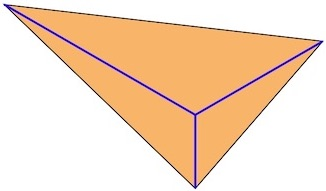# Magic Wye Triangles

Let a "Wye triangle" from lengths a, b, c be the triangle made by forming the letter Y, where the angles at the center are all 120° and a, b, c are the lengths of the three rays.

For example, the Wye triangle for 1, 2, 3 looks like this:Find a 3 × 3 array of distinct positive integers so that the six Wye triangles obtained from the rows and columns all have the same area. Try to make the sum of the 9 integers as small as possible.

Source: Lee Sallows (Nijmegen, The Netherlands) and Adam Sztrebonski (Champaign, Illinois).

18 February 2015